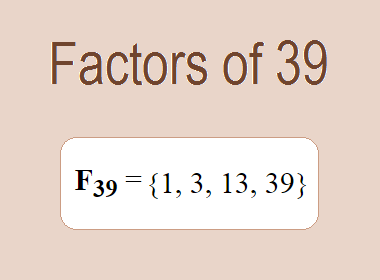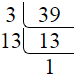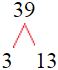# Factors of 39The factors of 39 are 1, 3, 13, and 39 i.e. F39 = {1, 3, 13, 39}. The factors of 39 are all the numbers that can divide 39 without leaving a remainder.

We can check if these numbers are factors of 39 by dividing 39 by each of them. If the result is a whole number, then the number is a factor of 39. Let's do this for each of the numbers listed above:

·        1 is a factor of 39 because 39 divided by 1 is 39.

·        3 is a factor of 39 because 39 divided by 3 is 13.

·        13 is a factor of 39 because 39 divided by 13 is 3.

·        39 is a factor of 39 because 39 divided by 39 is 1.

## How to Find Factors of 39?

1 and the number itself are the factors of every number. So, 1 and 39 are two factors of 39. To find the other factors of 39, we can start by dividing 39 by the numbers between 1 and 39. If we divide 39 by 2, we get a remainder of 1. Therefore, 2 is not a factor of 39. If we divide 39 by 3, we get a remainder of 0. Therefore, 3 is a factor of 39.

Next, we can check if 4 is a factor of 39. If we divide 39 by 4, we get a remainder of 3. Therefore, 4 is not a factor of 39. We can continue this process for all the possible factors of 39.

Through this process, we can find that the factors of 39 are 1, 3, 13, and 39. These are the only numbers that can divide 39 without leaving a remainder.

********************

********************

## Properties of the Factors of 39

The factors of 39 have some interesting properties. One of the properties is that the sum of the factors of 39 is equal to 56. We can see this by adding all the factors of 39 together:

1 + 3 + 13 + 39 = 56

Another property of the factors of 39 is that they are all odd numbers. This is because 39 is an odd number, and any even number cannot divide an odd number without leaving a remainder.

Another property of the factors of 39 is that the prime factors of 39 are 3 and 13.

## Applications of the Factors of 39

The factors of 39 have several applications in mathematics. One of the applications is in finding the highest common factor (HCF) of two or more numbers. The HCF is the largest factor that two or more numbers have in common. For example, to find the HCF of 39 and 52, we need to find the factors of both numbers and identify the largest factor they have in common. The factors of 39 are 1, 3, 13, and 39. The factors of 52 are 1, 2, 4, 13, 26, and 52. The largest factor that they have in common is 13. Therefore, the HCF of 39 and 52 is 13.

Another application of the factors of 39 is in prime factorization. Prime factorization is the process of expressing a number as the product of its prime factors. The prime factors of 39 are 3 and 13. We can express 39 as:

39 = 3 × 13

We can do prime factorization by division and factor tree method also. Here is the prime factorization of 39 by division method,39 = 3 × 13

Here is the prime factorization of 39 by the factor tree method,39 = 3 × 13

## Conclusion

The factors of 39 are the numbers that can divide 39 without leaving a remainder. The factors of 39 are 1, 3, 13, and 39. The factors of 39 have some interesting properties, such as being odd numbers and having a sum of 56. The factors of 39 have several applications in mathematics, such as finding the highest common factor and prime factorization.